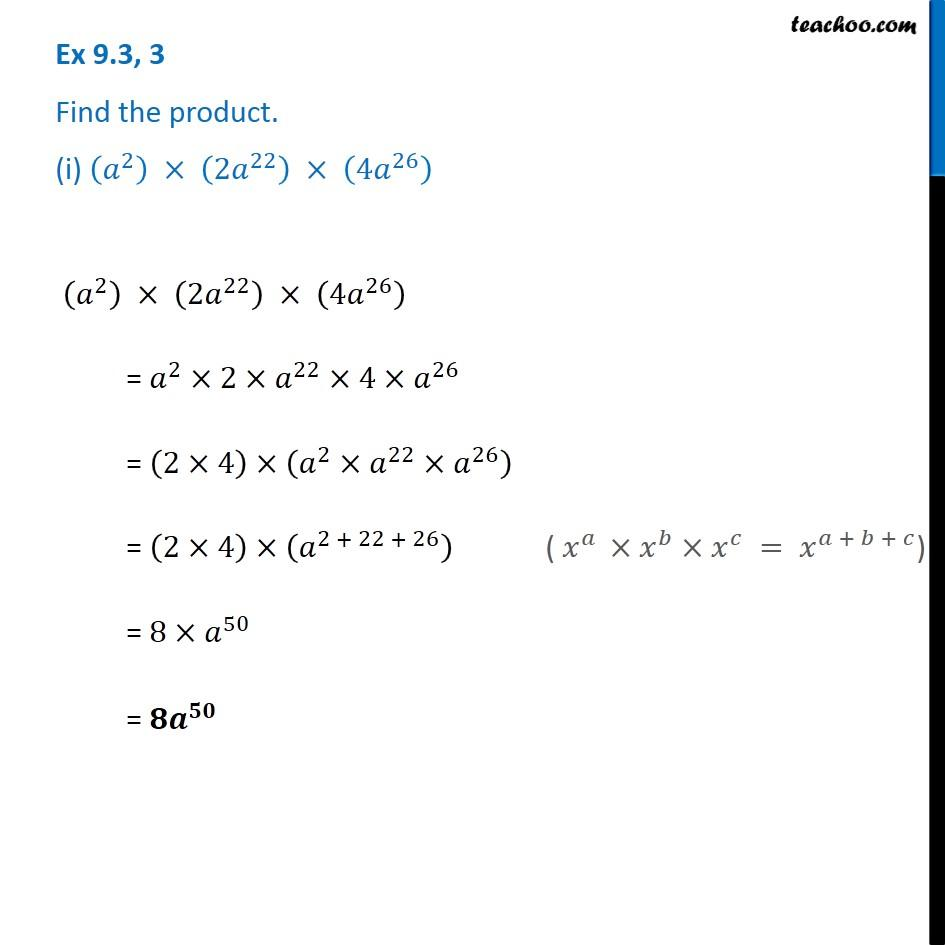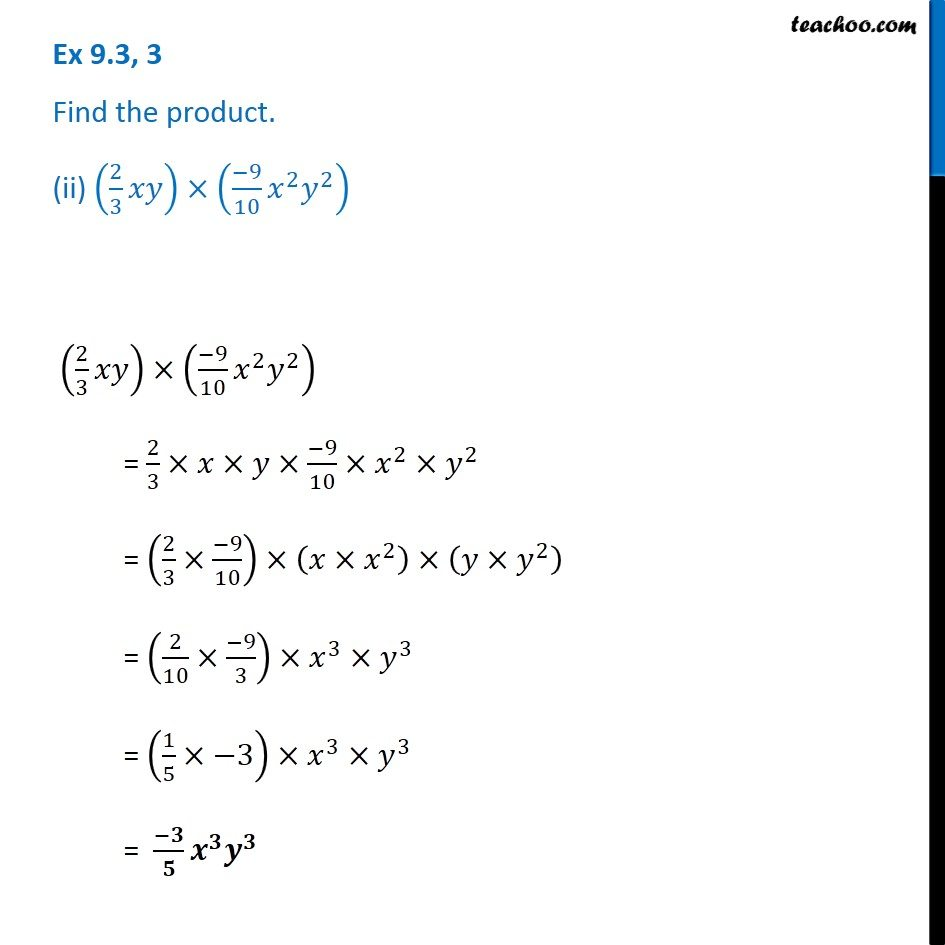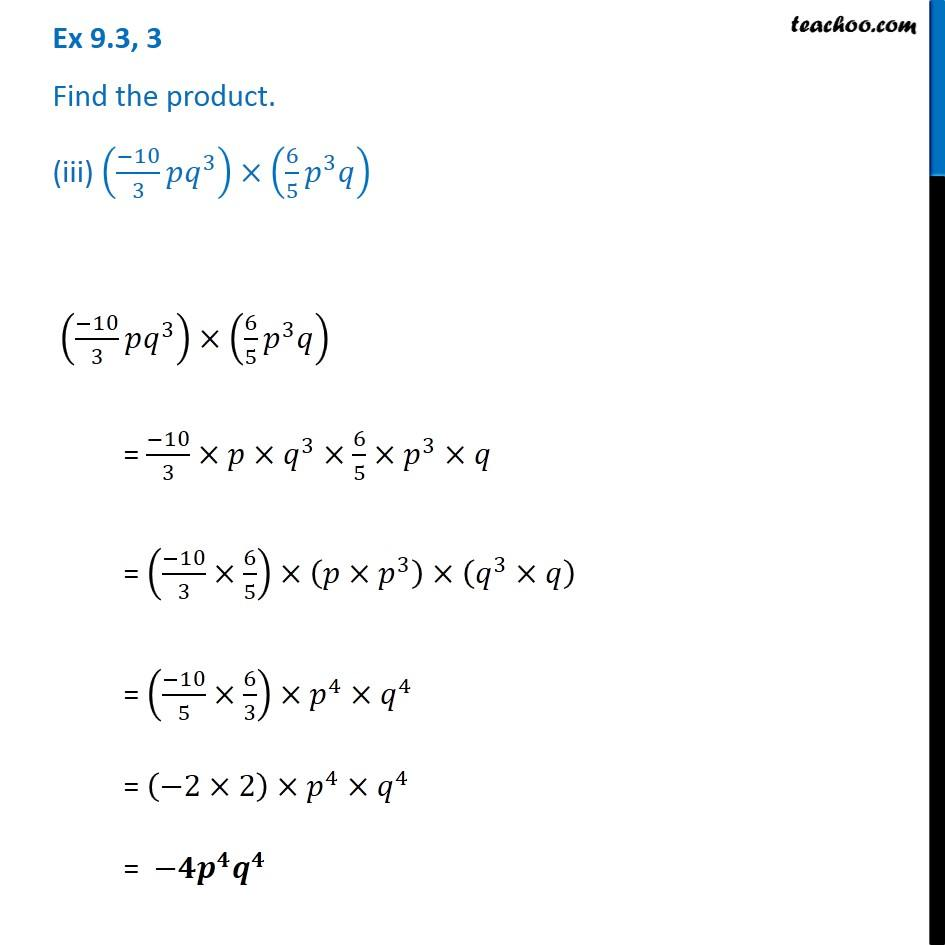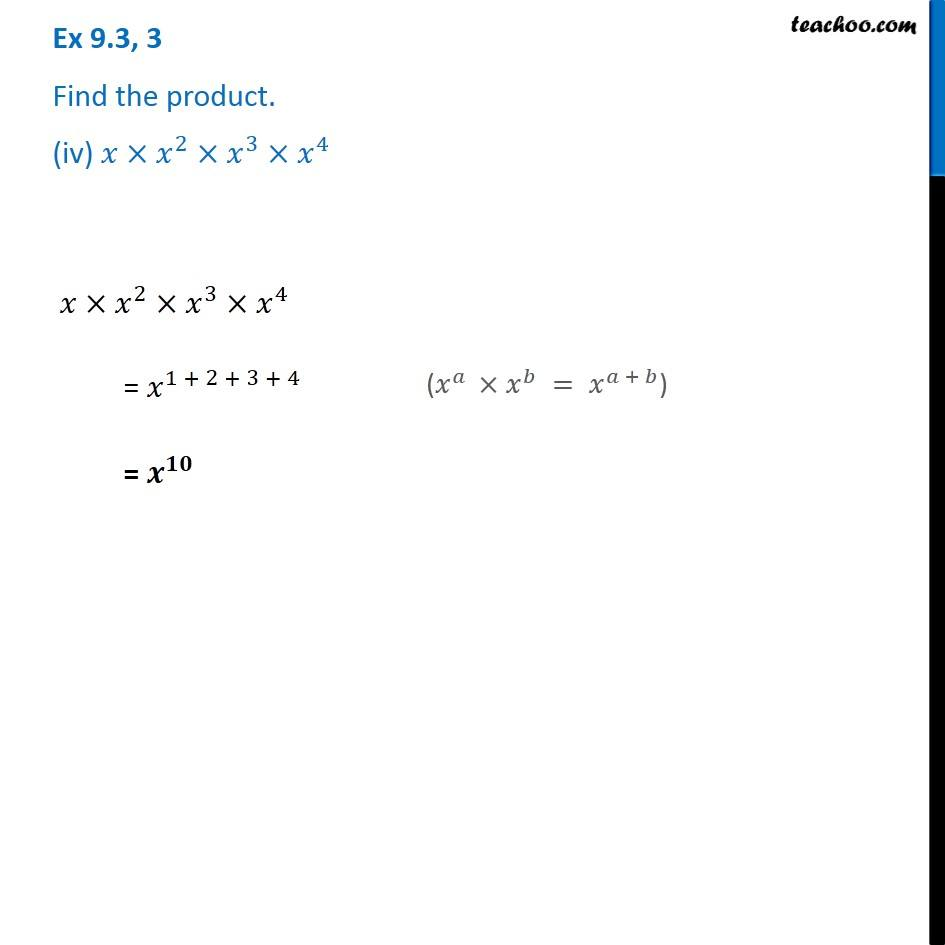Subscribe to our Youtube Channel - https://you.tube/teachoo

1. Chapter 9 Class 8 Algebraic Expressions and Identities
2. Serial order wise
3. Ex 9.3

Transcript

Ex 9.3, 3 Find the product. (i) (𝑎^2 ) × (2𝑎^22 ) × (4𝑎^26 ) (𝑎^2 ) × (2𝑎^22 ) × (4𝑎^26 ) = 𝑎^2×2×𝑎^22×4×𝑎^26 = (2×4)×(𝑎^2×𝑎^22×𝑎^26 ) = (2×4)×(𝑎^(2 + 22 + 26) ) ( 𝑥^𝑎 ×𝑥^𝑏×𝑥^𝑐 = 𝑥^(𝑎 + 𝑏 + 𝑐)) = 8×𝑎^50 = 𝟖𝒂^𝟓𝟎 Ex 9.3, 3 Find the product. (ii) (2/3 𝑥𝑦)×((−9)/10 𝑥^2 𝑦^2 ) (2/3 𝑥𝑦)×((−9)/10 𝑥^2 𝑦^2 ) = 2/3×𝑥×𝑦×(−9)/10×𝑥^2×𝑦^2 = (2/3×(−9)/10)×(𝑥×𝑥^2 )×(𝑦×𝑦^2 ) = (2/10×(−9)/3)×𝑥^3×𝑦^3 = (1/5×−3)×𝑥^3×𝑦^3 = (−𝟑)/𝟓 𝒙^𝟑 𝒚^𝟑 Ex 9.3, 3 Find the product. (iii) ((−10)/3 𝑝𝑞^3 )×(6/5 𝑝^3 𝑞) ((−10)/3 𝑝𝑞^3 )×(6/5 𝑝^3 𝑞) = (−10)/3×𝑝×𝑞^3×6/5×𝑝^3×𝑞 = ((−10)/3×6/5)×(𝑝×𝑝^3 )×(𝑞^3×𝑞) = ((−10)/5×6/3)×𝑝^4×𝑞^4 = (−2×2)×𝑝^4×𝑞^4 = −𝟒𝒑^𝟒 𝒒^𝟒 Ex 9.3, 3 Find the product. (iv) 𝑥×𝑥^2×𝑥^3×𝑥^4 𝑥×𝑥^2×𝑥^3×𝑥^4 = 𝑥^(1 + 2 + 3 + 4) = 𝒙^𝟏𝟎 (𝑥^𝑎 ×𝑥^𝑏 = 𝑥^(𝑎 + 𝑏))

Ex 9.3